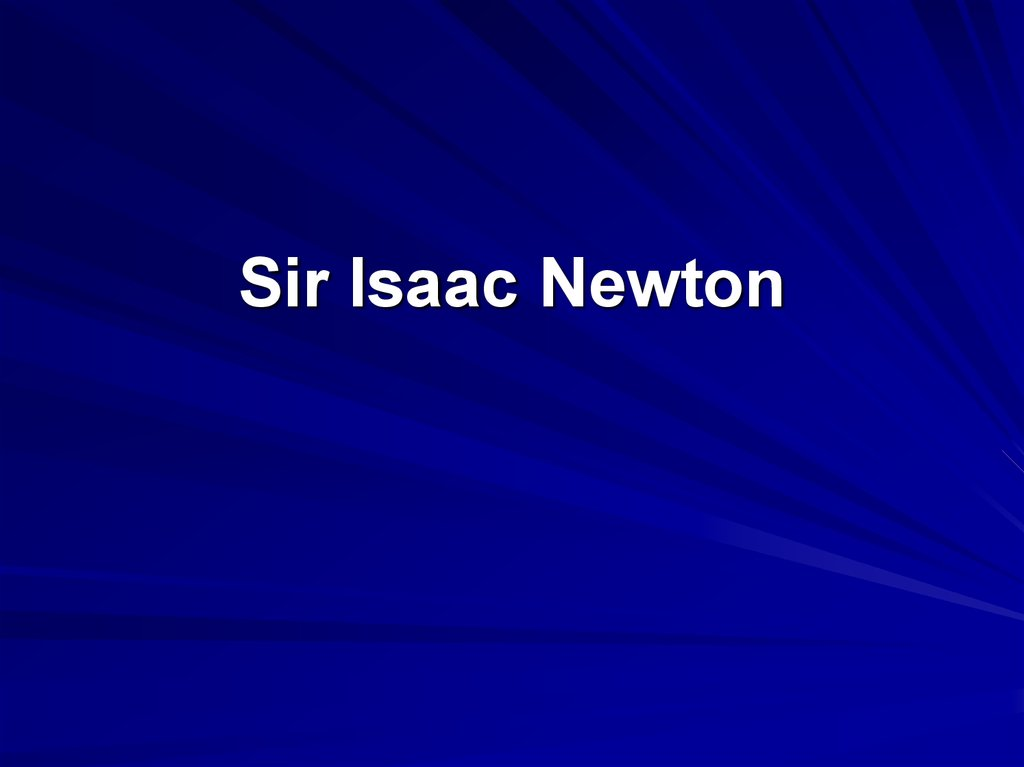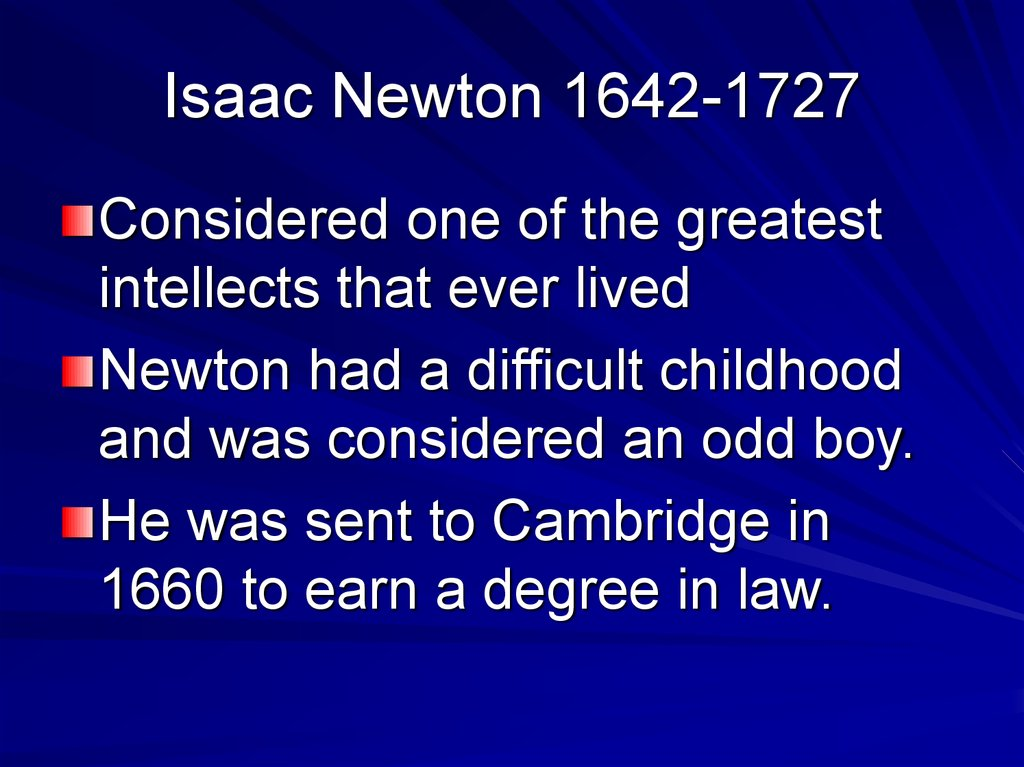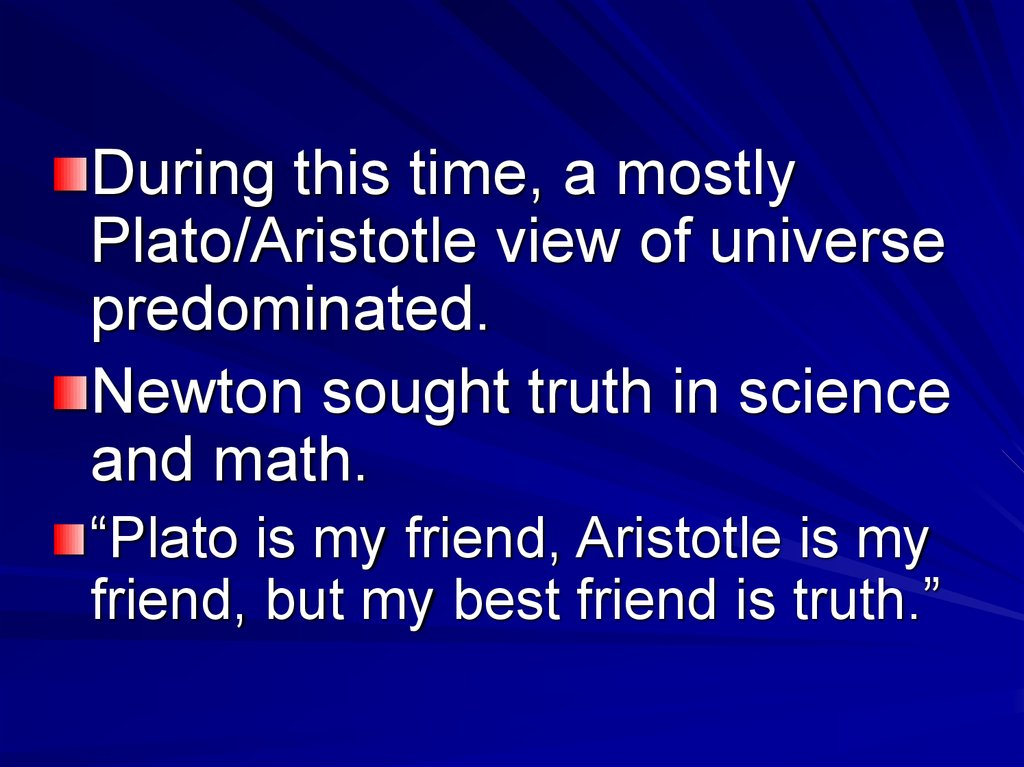# Isaac Newton 1642-1727

## 2. Isaac Newton 1642-1727

Considered one of the greatest
intellects that ever lived
Newton had a difficult childhood
and was considered an odd boy.
He was sent to Cambridge in
1660 to earn a degree in law.

## 3.

During this time, a mostly
Plato/Aristotle view of universe
predominated.
Newton sought truth in science
and math.
“Plato is my friend, Aristotle is my
friend, but my best friend is truth.”

## 4. Newton returns home

Graduated from Cambridge in
1665 with no distinction.
The plague closed university that
same year.
Newton returned to his family’s
farm for 18 months.

## 5. 1666 – Newton’s miracle year

Newton developed a theory of
light (white light is composed of
all colors).
He developed calculus.
Created his laws of motion.
And finally, created his universal
theory of gravitation.

## 6. Development of Calculus

He named it the “Method of
Fluxions.”
Used it for finding areas,
tangents, the lengths of curves
and the maxima and minima of
functions.

## 7.

Newton needed this to develop
his laws of motion and law of
gravitation.
The credit for calculus is now
shared with Leibniz.

## 8. Newton’s three laws of motion First Law

An object does what it’s already
doing unless affected by an
unbalanced force.
This is Galileo's concept of inertia.
Also called the “law of inertia”.
Friction is a force.

## 9.

This was more advanced than the
Plutonian view of movement.

## 10. Second Law of Motion

F = ma
F = net (unbalanced) force in
Newtons
m = mass in kilograms
2
a = acceleration in m/sec

## 11.

He probably used Galileo's
experimental conclusions to
develop this equation.
This equation can be developed
from lab experiments.

## 12. Newton’s Third Law of Motion

For every action, there is an equal
and opposite reaction.
For every force, there is an equal
and opposite force.

## 13. Newton’s Universal Gravitation Theory

His “ah-ha!” experience started with
a falling apple- does the moon also
fall?
The same laws that apply to the
Earth also apply to the heavens.

## 14. Fg α m1m2/s2

F = force in Newtons
α read as “is proportional to”
m1 = mass of first object in Kg
m2 = mass of second object in
Kg
s2 = distance between object’s
center of gravity in meters

## 15. Final form of gravitation equation

Fg = Gm1m2/s2
G = the gravitational constant
(determined 100 years after
Newton)
G = (6.67 x 10-11 N(m2/Kg2).

## 16.

Newton developed a
mathematical “proof” based on his
first 3 laws of motion.
This law also supported by
Kepler’s calculations and orbit
data.

## 17. New paradigm for society

The universe is subject to “cause
and effect” (responding to forces).
Less magic, and more reason.
All of nature can be explained by
math and reason.
The universe is like a giant windup clock, set in motion at creation.

## 18. Newton’s Social Life

Became a member of Royal
Society of Science in 1672.
Reluctant to publish, because he
was sensitive to criticism.
Was also very vindictive to
enemies.

## 19. Strengths of Newton’s Theory

Explains almost all observations.
Can accurately calculate
outcomes (used to calculate
everything from space shuttles to
the design of skyscrapers).
For the most part, is derived by
applying logic to everyday
experience (applied common
sense).

## 20. Weaknesses of Newton’s Theory

The theory did not explain the
cause of gravity – Why does
mass attract mass?
Eventually, the absolute speed of
light would create problems.